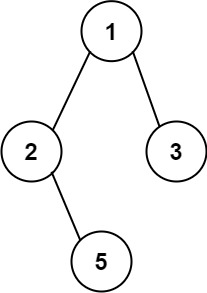GeetCode Hub

Given the root of a binary tree, return all root-to-leaf paths in any order.

A leaf is a node with no children.

Example 1:Input: root = [1,2,3,null,5]
Output: ["1->2->5","1->3"]

Example 2:

Input: root = 
Output: ["1"]

Constraints:

• The number of nodes in the tree is in the range [1, 100].
• -100 <= Node.val <= 100

/** * Definition for a binary tree node. * public class TreeNode { * int val; * TreeNode left; * TreeNode right; * TreeNode() {} * TreeNode(int val) { this.val = val; } * TreeNode(int val, TreeNode left, TreeNode right) { * this.val = val; * this.left = left; * this.right = right; * } * } */ class Solution { public List<String> binaryTreePaths(TreeNode root) { } }